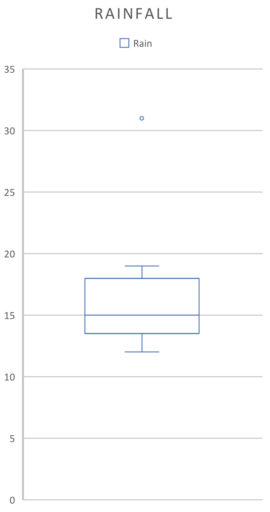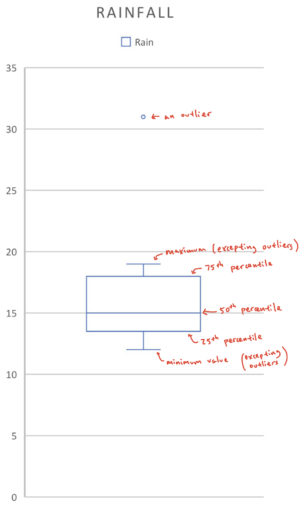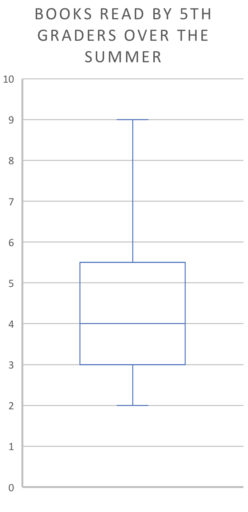A box plot is so named for its iconic shape:(They can also be laid out horizontally). The parts of the graph correspond to:Looking at a box plot can give you a quick sense of what the distribution of the data looks like. For example:

Example 1

Find the 25th percentile, 50th percentile, 75th percentile, range, and median for the below boxplot:Example 2

The following chart represents the books read by the 500 fifth-graders in a school over the summer. Suppose no student read exactly 4 books. How many students read more than 4 books?Example 3

The following chart represents the books read by the 500 fifth-graders in a school over the summer. What is the approximate number of students that have read between 3 and 4 books?Practice Problems

1. The following chart represents the books read by the 100 fifth-graders in a school over the summer. What is the approximate number of students that have read more than 5 books? (We assume that people can only read positive integers of books).2. The following chart represents the books read by the 500 fifth-graders in a school over the summer. Suppose 7 is the 80th percentile of this data. Approximately how many people read between 6 and 7 books? (We assume that people can only read positive integers of books).3. Researchers measured, over 36 months, how often it rained in a month. About how many months had rain from between 13 and 18 days?